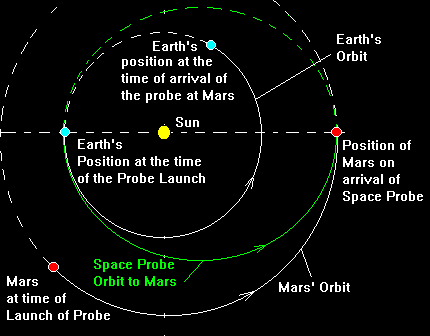## Orbital Transfers between Planetary Orbits:An ExampleWe will:

1. Determine the orbit Shape needed.
2. Determine the time of flight to the planet.
3. Determine the time when the space probe must be launched.
4. Determine the velocity the probe must have at launch to reach Mars.

The diagram shows the geometry of the orbits in our example. The orbit of the space probe must have perihelion at the Earth's Orbit and aphelion at the orbit of the target planet. This allows us to determine the shape and the semi-major axis of the orbit.

Let's use the example of sending a probe to Mars.

• rperihelion= Earth orbital radius = 1 AU
• raphelion= Mars orbital radius = 1.524 AU
• a = semimajor axis of probe orbit = (rperihelion+ raphelion)/2
a = (1 + 1.524)/2 = 1.262 AU
• The eccentricity of the transfer orbit is found from the fact that
r(perihelion) = a (1 - e)
eccentricity = e = 1 - rperihelion/a = 1 - 1/1.262 = 0.208
• We now can use Kepler's third law to determine the orbital period, P.
P2 = a3
period = (1.262)3/2 = 1.418 years = 518 days
• Since the probe takes half of a period to get to Mars, the time of flight is 259 days or 0.709 years.
• This means that the probe must be launched 261 days before Mars is at its position in its orbit where the probe will intercept Mars. Mars' orbital period is (1.524)3/2 = 1.88 years and it will move 136 degrees in its orbit during the probe's trip to Mars. Of course the Earth will have moved 0.709*360 = 255 degrees in its orbit during this time.

When should the launch take place? At the time of launch Mars must be (180-136)=44 degrees greater heliocentric longitude than the Earth.

• The relative rate of motion is needed to determine how long it takes Earth to make up
• those 44 degrees and be at opposition with Mars.
• The Earth moves in its orbit at 360/365.25 = 0.986 degrees/day
• Mars' orbital period is 1.88 times that of the Earth so Mars move slower and at a rate of 1/188*(0.986) = 0.524 degrees/day
• The difference in these two rates is 0.986-0.524 = 0.462 degrees/day
• This means that Earth will catch up to Mars in (44 deg)/(0.462 deg/day) = 96 days
• The launch should take place 96 day before opposition with Mars.

• With a little more physics we could perform calculations that would allows to determine the speeds needed by the probe to get to Mars. The Earth is already going at 29.7 km/s in its orbit and so provides part of the speed needed. This is the reason the probe is put into orbit in the counter clockwise direction : the same direction that the Earth and Mars are going around the Sun. The orbit we have calculated can be shown to be the lowest energy orbit needed to get directly from Earth to Mars.

At What Speed Should the Space Probe be Launched from Earth Orbit?

• We must use Kepler's second law to be able to calculate this quantity. In general terms it is not easy to express this law quantitatively. However, at perihelion and aphelion, it is easy.
• The relative velocities are inversely proportion to the respective distances from the sun.
rperihelionvperihelion = raphelionvaphelion
• We will not a derivation with this but with only geometry of ellipses, one can show that the two velocities are given as:
• vperihelion = vcircular[(1+e)/(1-e)]1/2
• vaphelion = vcircular[(1-e)/(1-e)]1/2
• The circular velocity is the average velocity in orbit = 2pa/P
where P = period of the orbit
• The Space Probe's circular velocity = 2*(3.1416)*(1.262AU)/(1.418 years) = 5.59 AU/yr
• Orbital velocities are usually expressed in kilometres/second.
The conversion constant is 4.73 km/s per AU/yr.
• v(perihelion) = 5.59*4.73*[(1+0.208)/(1-0.208)]1/2 = 32.7 km/s
• Since the Earth is moving at 29.7 km/s in its orbit, the velocity of the probe must be increased by 3.0 km/s relative to the Earth. (NOTE: This ignores the pull of the Earth's gravity and is true when the probe is outside the influence of Earth's gravity)
• What speed will the space probe have at Mars?Following the calculations above, you should be able to calculate how much the space probe must be slowed to be at the same velocity as Mars at rendevous. Calculate the speed of Mars in its orbit. Calculate the probes velocity at aphelion. The difference in the two is the amount that the prove must be slowed. Try it. You should find it must be slowed by about 2.7 km/s = 9630 km/hr